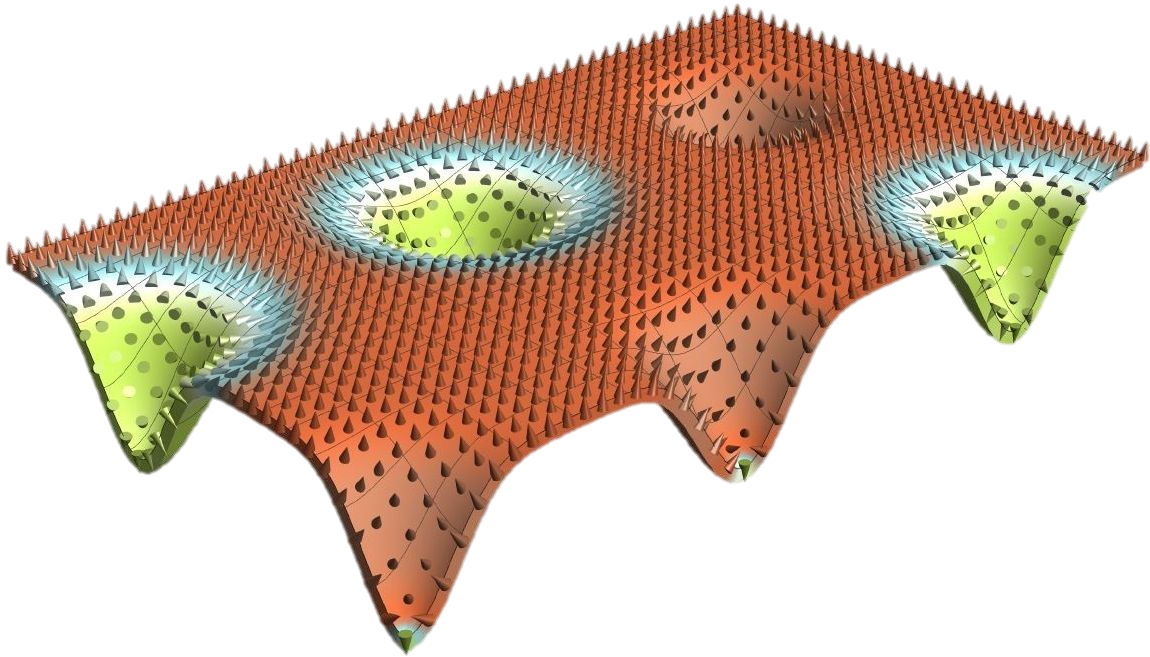Multiplet of Skyrmion States on a Curvilinear Defect: Reconfigurable Skyrmion Lattices

Typically, the chiral magnetic Skyrmion is a single-state excitation. Here we propose a system, where multiplet of Skyrmion states appears and one of these states can be the ground one. We show that the presence of a localized curvilinear defect drastically changes the magnetic properties of a thin perpendicularly magnetized ferromagnetic film. For a large enough defect amplitude a discrete set of equilibrium magnetization states appears forming a ladder of energy levels. Each equilibrium state has either a zero or a unit topological charge; i.e., topologically trivial and Skyrmion multiplets generally appear. Transitions between the levels with the same topological charge are allowed and can be utilized to encode and switch a bit of information. There is a wide range of geometrical and material parameters, where the Skyrmion level has the lowest energy. Thus, periodically arranged curvilinear defects can result in a Skyrmion lattice as the ground state.

Phys. Rev. Lett. 120 (2018), 067201, PDF
Supplementary Information